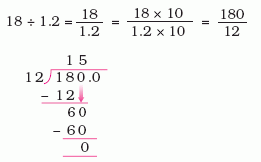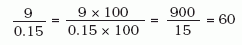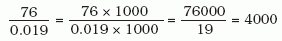Email us to get an instant 20% discount on highly effective K-12 Math & English kwizNET Programs!

#### Online Quiz (WorksheetABCD)

Questions Per Quiz = 2 4 6 8 10

### Grade 5 - Mathematics4.8 Dividing a Whole number by Decimal

Method:
• First we remove the decimal point from the denominator by multiplying both numerator and denominator with 10, 100 or 1000 etc.
• Perform the operation of division as usual.
 Divide 18 by 1.2 Here the dividend is a whole number and divisor is a 1 place decimal. We have to convert the divisor into a whole number by moving the decimal point to perform division.Divide 26 by 3.25 Here the dividend is a whole number and divisor is a 2 place decimal. We have to convert the divisor into a whole number by moving the decimal point to perform division.Divide 9 by 0.15Divide 76 by 0.019Directions: Divide the following whole number by decimals and find the quotient. Also write at least 5 examples of your own.
 Q 1: 144 ÷ 0.12Answer: Q 2: 42 ÷ 0.025Answer: Q 3: 804÷ 4.02Answer: Q 4: 1391÷ 1.3Answer: Q 5: 7÷ 0.014Answer: Q 6: 608 ÷ 0.32Answer: Question 7: This question is available to subscribers only! Question 8: This question is available to subscribers only!